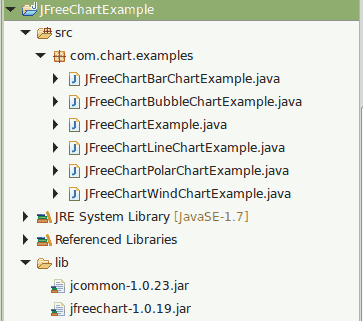# Create Area Chart Using JFreeChart

Create Area Chart Using JFreeChart explains about creating a simple area chart using JFreechart API

A area chart or area graph displays graphically quantitative data. It is based on the line chart. The area between axis and line are commonly emphasized with colors, textures and hatchings. Commonly one compares with an area chart two or more quantities.

Reference -> https://en.wikipedia.org/wiki/Area_chart

How To Create Area Chart Using JFreeChart library?

JFreeChart is a free and open source java chart library used for creating professional quality charts. JFreeChart is purely written in java language, we can very easily incorporate JFreeChart in our java standalone and web applications.

Referenece - > http://www.jfree.org/jfreechart/

Here I am showing an example about How to create  a simple Area Chart using JFreeChart.

Note

1) If you need pie chart example, You can follow Create Pie Chart Using JFreeChart

2) If you need line chart example, You can follow Create Line Chart Using JFreeChart

3) If you need bar chart example, You can follow Create Bar Chart Using JFreeChart

4) If you need polar chart example, You can follow Create Polar Chart Using JFreeChart

5) If you need bubble chart example, You can follow Create Bubble Chart Using JFreeChart

6) If you need wind chart example, You can follow Create Wind Chart Using JFreeChart

###### Required Libraries

Following jar must be in classpath

1. jfreechart-1.0.19.jar
2. jcommon-1.0.23.jar

We can create following professional quality charts by using jfreechart.

• Single valued Charts such as compass, speedometer, thermometer
• Line Chart
• Pie Chart
• Bar Chart
• Bubble Chart
• Wind Chart
• Polar Chart

## Project Structure